# Use of CountIfs Function in Microsoft Excel 2010

In this article, we will learn how to Count cells by range in Excel.

In simple words, while working with table values, sometimes we need to count the values that lays between the two given values. Example if we need to find the count of a certain IDs where the number corresponding to ID value must lay between given range. Criteria inside the formula executed using the operators.

If we need to count based on multiple criteria we should use the “COUNTIFS” function in Microsoft Excel 2010.

How to solve the problem ?

For this article we will be required to use the COUNTIFS function. Now we will make a formula out of the function. Here we are given some values in a range and specific range as criteria. We need to count the values where the number lay between given criteria.

Generic formula:-

 = COUNTIFS ( list , ">=low", list , "

list : list of numbers

low : lower limit of the given range (included)

high : higher limit of the given range (excluded)

Example :

Let’s take an example to understand how we can use this function.

Example 1: We have data in the range A1:C28,in which column A contains Dates, column B “Name”, column C “Revenue”. In column D we need to return the number of times revenue was collected by a person in a day.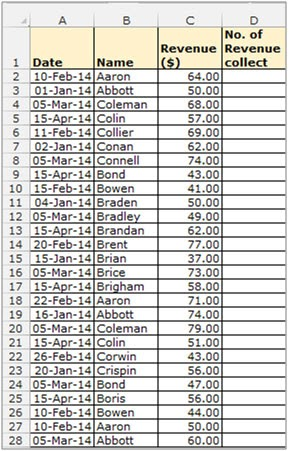• Select the Cell D2, and write the formula
• =COUNTIFS (\$A\$2:\$A\$28, A2, \$B\$2:\$B\$28, B2).
• Press Enter on the keyboard.
• The function will return the number. of times , that “Aaron” picked the revenue on 10th of February.
• To return the value for all candidates Copy the formula in all cells by pressing the key “CTRL + C” and select the cell D3 to D28 and press key “CTRL + V” on the keyboard.This function uses columns A & B as the criteria column. The result has to be obtained based on a match obtained in both columns.

All of these might be confusing to understand. So, let's test this formula via running it on the example shown below.

Here we have the ID records and we need to find the COUNT of ID values where respective numbers lays between the given range.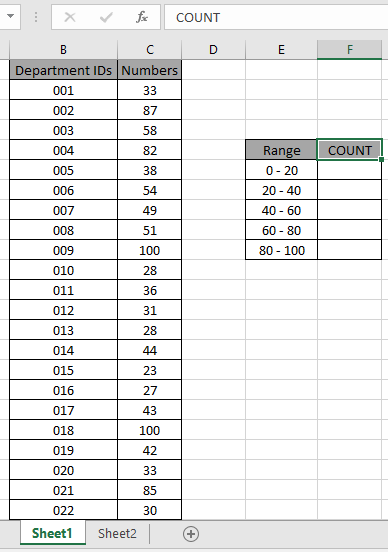Here to find the values in range. Some range references given using named range.

Numbers array given as Value named range for the array C3:C24.

Use the formula:

 = COUNTIFS ( Value , ">=0" , Value , "<20" )

Explanation:

• COUNTIFS function counts the cells that match the numbers in the Value array which are greater than equals to 0 (0 included).
• The COUNTIFS function counts the cells that match the numbers in the Value array which are less than 20 (20 excluded).
• The formula counts the cell which satisfies both conditions which means value which lay between 0 to 20 will be counted.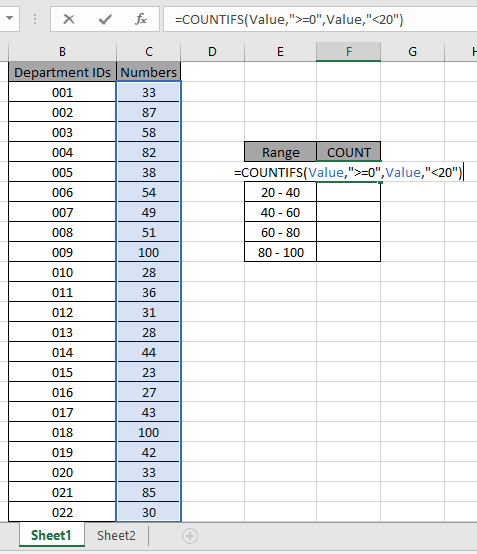The formula looks like as shown in the above snapshot. The Value array is given as a named range.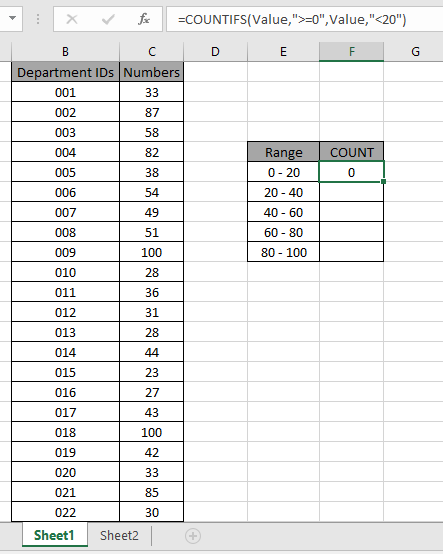As you can see the total values which occur between 0 - 20 comes out to be 0 i.e. there are no such values between 0 - 20.

Now copy the formula in other cells using the drag down option or using the shortcut key Ctrl + D as shown below. And changing the range parameter to 20 - 40.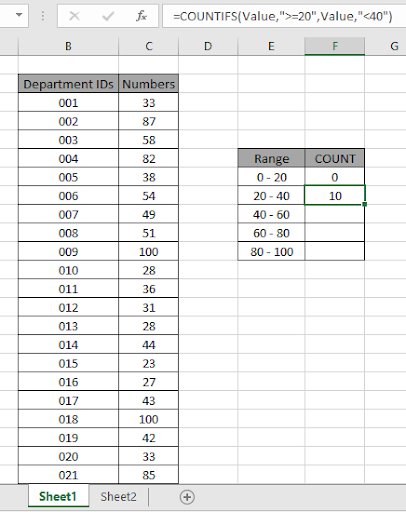As you can see values which lay between 20 - 40 are 10.

Now calculate all the other counts with given range values as explained above.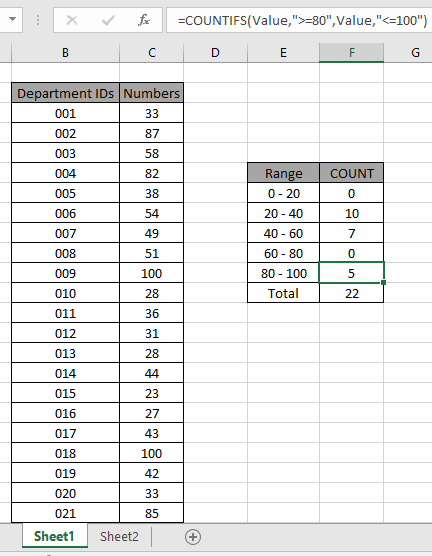We obtained all the count between given range as it also proves the formula works fine.

Here are some observational notes shown below.

Notes:

1. The formula only works with numbers and text both.
2. The formula ignores text value while comparing numbers and ignores numbers when match text values.
3. Operators like equals to ( = ), less than equal to ( <= ), greater than ( > ) or not equals to ( <> ) can be performed within a function applied with number only.

Hope this article about how to Count cells by range in Excel is explanatory. Find more articles on COUNTIFS functions here. If you liked our blogs, share it with your friends on Facebook. And also you can follow us on Twitter and Facebook. We would love to hear from you, do let us know how we can improve, complement or innovate our work and make it better for you. Write us at info@exceltip.com

Related Articles

How to use the SUMPRODUCT function in Excel: Returns the SUM after multiplication of values in multiple arrays in excel.

COUNTIFS with Dynamic Criteria Range : Count cells dependent on other cell values in Excel.

COUNTIFS Two Criteria Match : Count cells matching two different criteria on list in excel.

COUNTIFS With OR For Multiple Criteria : Count cells having multiple criteria match using the OR function.

The COUNTIFS Function in Excel : Count cells dependent on other cell values.

How to Use Countif in VBA in Microsoft Excel : Count cells using Visual Basic for Applications code.

How to use wildcards in excel : Count cells matching phrases using the wildcards in excel

Popular Articles :

How to use the IF Function in Excel : The IF statement in Excel checks the condition and returns a specific value if the condition is TRUE or returns another specific value if FALSE.

How to use the VLOOKUP Function in Excel : This is one of the most used and popular functions of excel that is used to lookup value from different ranges and sheets.

How to Use SUMIF Function in Excel : This is another dashboard essential function. This helps you sum up values on specific conditions.

How to use the COUNTIF Function in Excel : Count values with conditions using this amazing function. You don't need to filter your data to count specific values. Countif function is essential to prepare your dashboard.

Terms and Conditions of use

The applications/code on this site are distributed as is and without warranties or liability. In no event shall the owner of the copyrights, or the authors of the applications/code be liable for any loss of profit, any problems or any damage resulting from the use or evaluation of the applications/code.